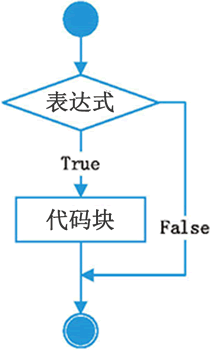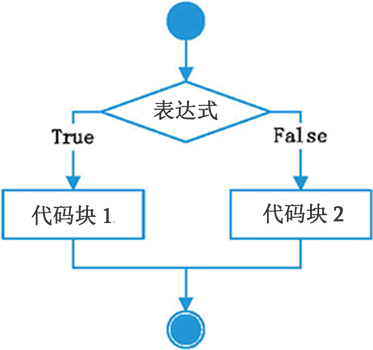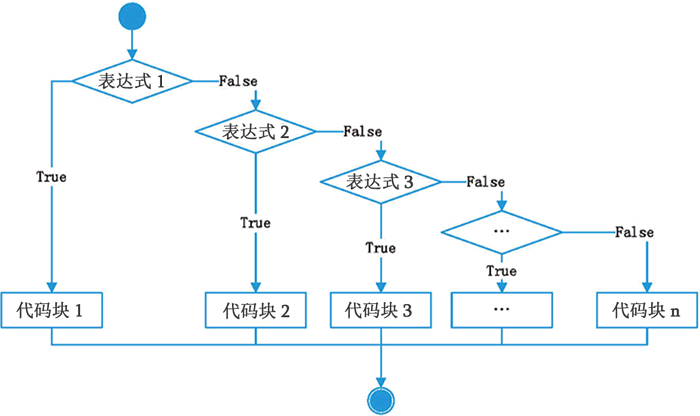## Python if else条件语句详解

• 内容
• 评论
• 相关

Python 中的 if else 语句可以细分为三种形式，分别是 if 语句、if else 语句和 if elif else 语句，它们的语法和执行流程如表1所示。

if 表达式：
代码块if 表达式：
代码块 1
else：
代码块 2if 表达式 1：
代码块 1
elif 表达式 2：
代码块 2
elif 表达式 3：
代码块 3
...//其它elif语句
else：
代码块 n• “表达式”可以是一个单一的值或者变量，也可以是由运算符组成的复杂语句，形式不限，只要它能得到一个值就行。不管“表达式”的结果是什么类型，if else 都能判断它是否成立（真或者假）。
• “代码块”由具由相同缩进量的若干条语句组成。
• if、elif、else 语句的最后都有冒号`:`，不要忘记。

【实例1】使用第一种选择结构判断用户是否符合条件：

```age = int( input("请输入你的年龄：") )

if age < 18 :
print("你还未成年，建议在家人陪同下使用该软件！")
print("如果你已经得到了家长的同意，请忽略以上提示。")

#该语句不属于if的代码块
print("软件正在使用中...")```

【实例2】改进上面的代码，年龄不符合时退出程序：

```import sys

age = int( input("请输入你的年龄：") )

if age < 18 :
print("警告：你还未成年，不能使用该软件！")
print("未成年人应该好好学习，读个好大学，报效祖国。")
sys.exit()
else:
print("你已经成年，可以使用该软件。")
print("时间宝贵，请不要在该软件上浪费太多时间。")

print("软件正在使用中...")```

sys 模块的 exit() 函数用于退出程序。

【实例3】判断一个人的身材是否合理：

```height = float(input("输入身高（米）："))
weight = float(input("输入体重（千克）："))
bmi = weight / (height * height)  #计算BMI指数

if bmi<18.5:
print("BMI指数为："+str(bmi))
print("体重过轻")
elif bmi>=18.5 and bmi<24.9:
print("BMI指数为："+str(bmi))
print("正常范围，注意保持")
elif bmi>=24.9 and bmi<29.9:
print("BMI指数为："+str(bmi))
print("体重过重")
else:
print("BMI指数为："+str(bmi))
print("肥胖")```

BMI指数为：24.221453287197235

## if else 如何判断表达式是否成立

""  #空字符串
[ ]  #空列表
( )  #空元组
{ }  #空字典
None  #空值

【实例】if elif 判断各种类型的表达式：

```b = False
if b:
print('b是True')
else:
print('b是False')

n = 0
if n:
print('n不是零值')
else:
print('n是零值')

s = ""
if s:
print('s不是空字符串')
else:
print('s是空字符串')

l = []
if l:
print('l不是空列表')
else:
print('l是空列表')

d = {}
if d:
print('d不是空字典')
else:
print('d是空字典')

def func():
print("函数被调用")

if func():
print('func()返回值不是空')
else:
print('func()返回值为空')```

b是False
n是零值
s是空字符串
l是空列表
d是空字典

func()返回值为空

0条评论